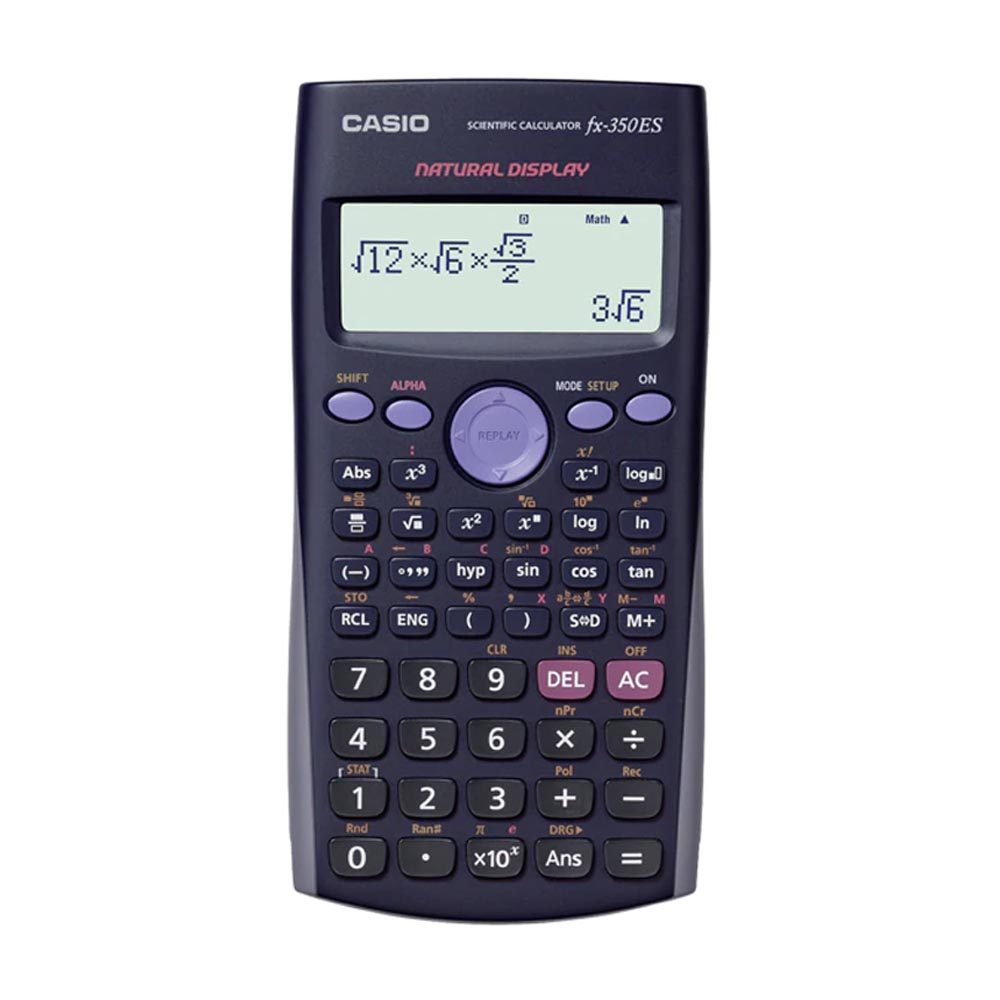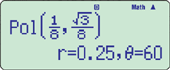# CASIO FX-350ES, SCIENTIFIC, CALCULATOR

• Brand: CASIO
• Product SKU: WH05035
• Availability: instock
• Delivery: Within 3 working days
AED58 AED 54

.

Features

New functions
• Random integers
• Prime factorizations
Standard functions
• 9 variables
• Table function
• Fraction calculations
• Combination and permutation
• Comes with new slide-on hard case
• Statistics (List-based STAT data editor, standard deviation, regression analysis)
• #### Coordinate transformation• #### Power calculation#### Statistics

•••#### Trigonometry

•#### Table function

•••••••Specifications

• Non Programmable

•Number of Functions : 252

•AAA ï¿½ 1 (LR03)

•Natural textbook display
Display expression same as textbook.

•10 + 2 digits
10-digit mantissa + 2-digit exponential display.

•Dot matrix display
High-resolution screen provides beautiful looking graphs every time.

•Multi-replay
Quick and easy recall of previously executed formulas for editing and re-execution.

•Plastic keys
Designed and engineered for easy operation.

• Table Generation
You can create a numerical table based on that expression by registering a function expression. In addition, graph functions can be represented by graphs.

• Basic Mathematical Functions
Trigonometric, Exponential logarithmic, etc.

• Basic Statistics
Standard statistics functions such as Mean, SUM, Standard Deviation, and Regression

• AAA-size Battery

• Non Graphing

•List based STAT-data editor
Viewing and editing of input data in list format, showing data groups (x-data, y-data, frequency) and surrounding data.

Top